# Remainder

What is the remainder of the division of natural numbers 293 and 7?

z =  6

### Step-by-step explanation: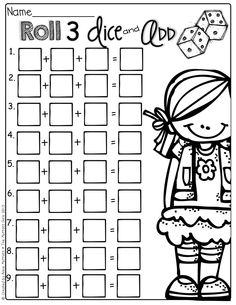Did you find an error or inaccuracy? Feel free to write us. Thank you!Tips to related online calculators
Do you want to round the number?
Do you want to perform natural numbers division - find the quotient and remainder?

## Related math problems and questions:

• RemaindersIt is given a set of numbers { 170; 244; 299; 333; 351; 391; 423; 644 }. Divide this numbers by number 66 and determine set of remainders. As result write sum of this remainders.
• On Children'sOn Children's Day, the organizers bought 252 chewing gums, 396 candies and 108 lollipops. They want to make as many of the same packages as possible. Advise them what to put in each package and how many packages they can make this way.
• Quotient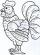Determine the quotient (q) and the remainder (r) from division numbers 100 and 8. Take the test of correctness.
• Cash back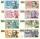After a trip 24 students have left more than 650 but less than 690 CZK (Kc). How much each student receives return when the amount is divisible by the number of pupils?
• How manyHow many integers are greater than 547/3 and less than 931/4?
• Reminder and quotientThere are given numbers A = 135, B = 315. Find the smallest natural number R greater than 1 so that the proportions R:A, R:B are with the remainder 1.
• Farmer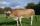The farmer farming 293 ha of arable land and the remaining 20% are meadows and pastures. How much land farmer owns?
• RemainderA is an arbitrary integer that gives remainder 1 in the division with 6. B is an arbitrary integer that gives remainder 2 the division by. What makes remainder in division by 3 product of numbers A x B ?
• Promile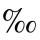Calculate the 4.6 ‰ of 199.
• Divisible by nineHow many three-digit natural numbers in total are divisible without a remainder by the number 9?
• Simple equation 2Find X in this simple equation: X/9 = 96/108
• Coloured numbersMussel wrote four different natural numbers with coloured markers: red, blue, green and yellow. When the red number divides by blue, it gets the green number as an incomplete proportion, and yellow represents the remainder after this division. When it div
• Result and remainderAfter dividing the unknown number by the number 23, the quotient 11 and the remainder 4 are formed. Find an unknown number.
• Simple sequence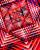Continue with this series of numbers: 1792,448, 112, _, _
• Statistics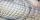The sum of all deviations from the arithmetic mean of the numerical sequence 4, 6, 51, 77, 90, 93, 95, 109, 113, 117 is:
• School year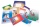At the beginning of the school year, 396 notebooks and 252 textbooks are ready to be distributed in the classroom. All pupils receive the same number of notebooks and the same amount of textbooks. How many pupils are there in the class if you know that th
• Unknown integerFind the smallest integer: divided by 2, the remainder is 1. divided by 3, the remainder is 2. divided by 4, the remainder is 3. ... divided by eight, the remainder is 7, divided by 9 the remainder is 8.